# 5.2: Ring Strain and the Structure of Cycloalkanes

Objectives

After completing this section, you should be able to

1. describe the Baeyer strain theory.
2. describe how the measurement of heats of combustion provides information about the amount of strain present in a cycloalkane ring.
3. determine the relative stability of cyclic compounds, by assessing such factors as angle strain, torsional strain and steric strain.

Key Terms

Make certain that you can define, and use in context, the key terms below.

• angle strain
• steric strain
• torsional strain
• ring strain
• heat of combustion

## Heat of Combustion as a Measure of Bond Strength

The combustion of carbon compounds, especially hydrocarbons, has been the most important source of heat energy for human civilizations throughout recorded history. The practical importance of this reaction cannot be denied, but the massive and uncontrolled chemical changes that take place in combustion make it difficult to deduce mechanistic paths. Using the combustion of propane as an example, we see from the following equation that every covalent bond in the reactants has been broken and an entirely new set of covalent bonds have formed in the products. No other common reaction involves such a profound and pervasive change, and the mechanism of combustion is so complex that chemists are just beginning to explore and understand some of its elementary features.Since all the covalent bonds in the reactant molecules are broken, the quantity of heat evolved in this reaction, and any other combustion reaction, is related to the strength of these bonds (and, of course, the strength of the bonds formed in the products). Precise heat of combustion measurements can provide useful information about the structure of molecules and their relative stability.

For example, heat of combustion is useful in determining the relative stability of isomers. Pentane has a heat of combustion of -782 kcal/mol, while that of its isomer, 2,2-dimethylpropane (neopentane), is –777 kcal/mol. These values indicate that 2,3-dimethylpentane is 5 kcal/mol more stable than pentane, since it has a lower heat of combustion.

## Ring Strain

Table $$\PageIndex{1}$$ lists the heat of combustion data for some simple cycloalkanes. These cycloalkanes do not have the same molecular formula, so the heat of combustion per each CH2 unit present in each molecule is calculated (the fourth column) to provide a useful comparison. From the data, cyclopropane and cyclobutane have significantly higher heats of combustion per CH2, while cyclohexane has the lowest heat of combustion. This indicates that cyclohexane is more stable than cyclopropane and cyclobutane, and in fact, that cyclohexane has a same relative stability as long chain alkanes that are not cyclic. This difference in stability is seen in nature where six membered rings are by far the most common.  What causes the difference in stability or the strain in small cycloalkanes?

Table $$\PageIndex{1}$$: Heats of combustion of select hydrocarbons
Cycloalkane
(CH2)n
CH2 Units
n
ΔH25º
kcal/mol
ΔH25º
per CH2 Unit
Ring Strain
kcal/mol
Cyclopropane n = 3 468.7 156.2 27.6
Cyclobutane n = 4 614.3 153.6 26.4
Cyclopentane n = 5 741.5 148.3 6.5
Cyclohexane n = 6 882.1 147.0 0.0
Cycloheptane n = 7 1035.4 147.9 6.3
Cyclooctane n = 8 1186.0 148.2 9.6
Cyclononane n = 9 1335.0 148.3 11.7
Cyclodecane n = 10 1481 148.1 11.0
CH3(CH2)mCH3 m = large 147.0 0.0

## The Baeyer Theory on the Strain in Cycloalkane Rings

In 1890, the famous German organic chemist, A. Baeyer, suggested that cyclopropane and cyclobutane are less stable than cyclohexane, because the the smaller rings are more "strained". There are many different types of strain that contribute to the overall ring strain in cycloalkanes, including angle strain, torsional strain, and steric strain. Torsional strain and steric strain were previously defined in the discussion of conformations of butane. Angle Strain occurs when the sp3 hybridized carbons in cycloalkanes do not have the expected ideal bond angle of 109.5o, causing an increase in the potential energy. An example of angle strain can be seen in the diagram of cyclopropane below in which the bond angle is 60o between the carbons.  The compressed bond angles causes poor overlap of the hybrid orbitals forming the carbon-carbon sigma bonds which in turn creates destabilization.The C-C-C bond angles in cyclopropane (diagram above) (60o) and cyclobutane (90o) are much different than the ideal bond angle of 109.5o. This bond angle causes cyclopropane and cyclobutane to be less stable than molecules such as cyclohexane and cyclopentane, which have a much lower ring strain because the bond angle between the carbons is much closer to 109.5o. Changes in chemical reactivity as a consequence of angle strain are dramatic in the case of cyclopropane, and are also evident for cyclobutane.

In addition to angle strain, there is also steric (transannular) strain and torsional strain in many cycloalkanes. Transannular strain exists when there is steric repulsion between atoms.Because cycloalkane lack the ability to freely rotate, torsional (eclipsing) strain exists when a cycloalkane is unable to adopt a staggered conformation around a C-C bond. Torsional strain is especially prevalent in small cycloalkanes, such as cyclopropane, whose structures are nearly planar.The Eclipsed C-H Bonds in Cyclopropane

Larger rings like cyclohexane, deal with torsional strain by forming conformers in which the rings are not planar. A conformer is a stereoisomer in which molecules of the same connectivity and formula exist as different isomers, in this case, to reduce ring strain. The ring strain is reduced in conformers due to the rotations around the sigma bonds, which decreases the angle and torsional strain in the ring. The non-planar structures of cyclohexane are very stable compared to cyclopropane and cyclobutane, and will be discussed in more detail in the next section.Angle Strain The strain caused by the increase or reduction of bond angles Torsional Strain The strain caused by eclipsing bonds on adjacent atoms Steric Strain The strain caused by the repulsive interactions of atoms trying to occupy the same space

Exercise $$\PageIndex{1}$$

trans-1,2-Dimethylcyclobutane is more stable than cis-1,2-dimethylcyclobutane. Explain this observation.

The trans form does not have eclipsing methyl groups, therefore lowering the energy within the molecule. It does however have hydrogen-methyl eclipsing interactions which are not as high in energy as methyl-methyl interactions.

Exercise $$\PageIndex{2}$$

Cyclobutane has more torsional stain than cyclopropane.  Explain this observation.

Cyclobutane has 4 CH2 groups while cyclopropane only has 3.  More CH2 groups means cyclobutane has more eclipsing H-H interactions and therefore has more torsional strain.

#### Questions

Q4.3.1

trans-1,2-Dimethylcyclobutane is more stable than cis-1,2-dimethylcyclobutane. Explain this observation.

#### Solutions

S4.3.1

The trans form does not have eclipsing methyl groups, therefore lowering the energy within the molecule. It does however have hydrogen-methyl interactions, but are not as high in energy than methyl-methyl interactions.

### Contributors

• William Reusch, Professor Emeritus (Michigan State U.), Virtual Textbook of Organic Chemistry
• Kelly Matthews, Senior Professor of Chemistry (Harrisburg Area Community College)
• Steven Farmer, Professor of Chemistry (Sonoma State University)

Objectives

After completing this section, you should be able to

1. describe, and sketch the conformation of cyclopropane, cyclobutane, and cyclopentane.
2. describe the bonding in cyclopropane, and use this to account for the high reactivity of this compound.
3. analyze the stability of cyclobutane, cyclopentane and their substituted derivatives in terms of angular strain, torsional strain and steric interactions.

Study Notes

Notice that in both cyclobutane and cyclopentane, torsional strain is reduced at the cost of increasing angular (angle) strain.

Although the customary line drawings of simple cycloalkanes are geometrical polygons, the actual shape of these compounds in most cases is very different.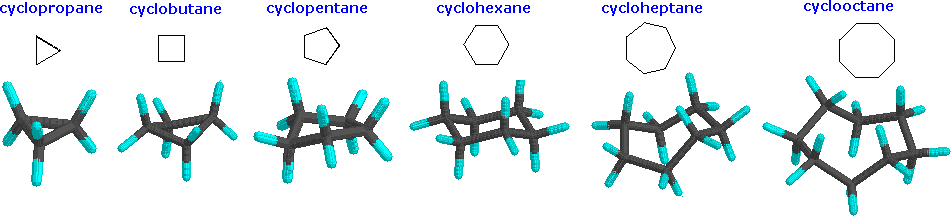Cyclopropane is necessarily planar (flat), with the carbon atoms at the corners of an equilateral triangle. The 60º bond angles are much smaller than the optimum 109.5º angles of a normal tetrahedral carbon atom, and the resulting angle strain dramatically influences the chemical behavior of this cycloalkane. Cyclopropane also suffers substantial eclipsing strain, since all the carbon-carbon bonds are fully eclipsed. Cyclobutane reduces some bond-eclipsing strain by folding (the out-of-plane dihedral angle is about 25º), but the total eclipsing and angle strain remains high. Cyclopentane has very little angle strain (the angles of a pentagon are 108º), but its eclipsing strain would be large (about 40 kJ/mol) if it remained planar. Consequently, the five-membered ring adopts non-planar puckered conformations whenever possible.

Rings larger than cyclopentane would have angle strain if they were planar. However, this strain, together with the eclipsing strain inherent in a planar structure, can be relieved by puckering the ring. Cyclohexane is a good example of a carbocyclic system that virtually eliminates eclipsing and angle strain by adopting non-planar conformations. Cycloheptane and cyclooctane have greater strain than cyclohexane, in large part due to transannular crowding (steric hindrance by groups on opposite sides of the ring).

Cyclic systems are a little different from open-chain systems. In an open chain, any bond can be rotated 360º, going through many different conformations. That complete rotation isn't possible in a cyclic system, because the parts that would be trying to twist away from each other would still be connected together. Thus cyclic systems have fewer "degrees of freedom" than aliphatic systems; they have "restricted rotation".

Because of the restricted rotation of cyclic systems, most of them have much more well-defined shapes than their aliphatic counterparts. Let's take a look at the basic shapes of some common rings. Many biologically important compounds are built around structures containing rings, so it's important that we become familiar with them. In nature, three- to six-membered rings are frequently encountered, so we'll focus on those.

## Cyclopropane

A three membered ring has no rotational freedom whatsoever. A plane is defined by three points, so the three carbon atoms in cyclopropane are all constrained to lie in the same plane. This lack of flexibility does not allow cyclopropane to form more stable conformers which are non-planar.

The main source of ring strain in cyclopropane is angle strain.  All of the carbon atoms in cyclopropane are tetrahedral and would prefer to have a bond angle of 109.5 The angles in an equilateral triangle are actually 60o, about half as large as the optimum angle. The large deviation from the optimal bond angle means that the C-C sigma bonds forming the cyclopropane ring are bent.  Maximum bonding occurs when the overlapping orbitals are pointing directly toward each other.  The severely strained bond angles in cyclopropane means that the orbitals forming the C-C bonds overlap at a slight angle making them weaker.  This strain is partially overcome by using so-called “banana bonds”, where the overlap between orbitals is no longer directly in a line between the two nuclei, as shown here in three representations of the bonding in cyclopropane: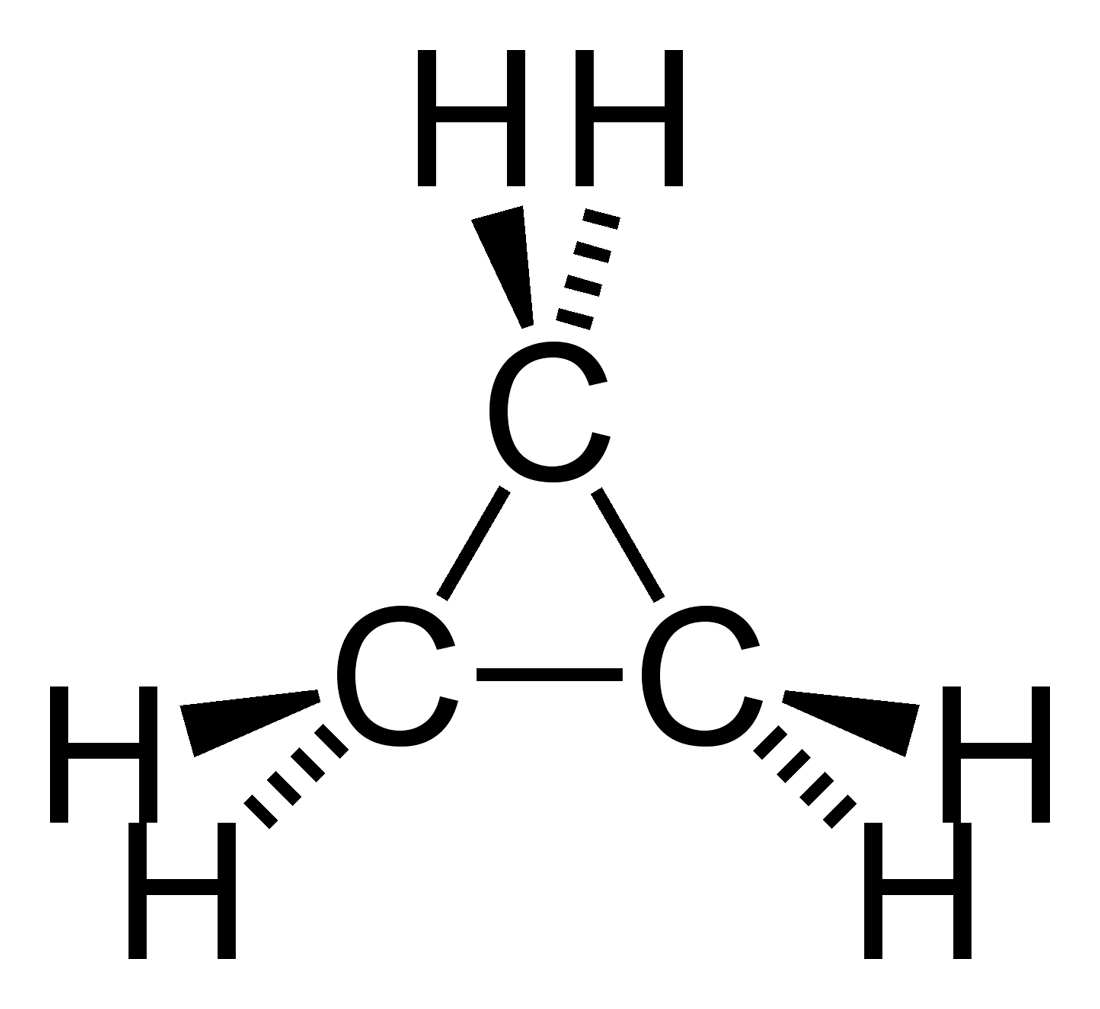The constrained nature of cyclopropane causes neighboring C-H bonds to all be held in eclipsed conformations. Cyclopropane is always at maximum torsional strain. This strain can be illustrated in a Newman projections of cyclopropane as shown from the side.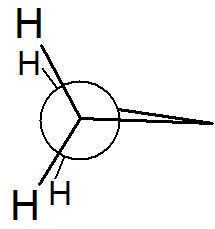Newman Projection of cyclopropane

Cyclopropane isn't large enough to introduce any steric strain. Steric strain does not become a factor until we reach six membered rings. Before that point, rings are not flexible enough to allow for two ring substituents to interact with each other.

Interactive Element

The 3D Structure of Cyclopropane

The combination of torsional and angle strain creates a large amount of ring strain in cyclopropane which weakens the C-C ring bonds (255 kJ/mol) when compared to C-C bonds in open-chain propane (370 kJ/mol).

## Cyclobutane

Cyclobutane is a four membered ring. The larger number of ring hydrogens would cause a substantial amount of torsional strain if cyclobutane were planar.In three dimensions, cyclobutane is flexible enough to buckle into a "puckered" shape which causes the C-H ring hydrogens to slightly deviate away from being completely eclipsed.  This conformation relives some of the torsional strain but increases the angle strain because the ring bond angles decreases to 88o.

In a line drawing, this butterfly shape is usually shown from the side, with the near edges drawn using darker lines.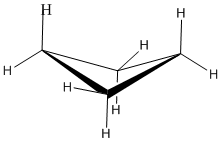The deviation of cyclobutane's ring C-H bonds away from being fully eclipsed can clearly be seen when viewing a Newman projections signed down one of the C-C bond.Newman projection of cyclobutane

• With bond angles of 88o rather than 109.5o degrees, cyclobutane has significant amounts of angle strain, but less than in cyclopropane.
• Although torsional strain is still present, the neighboring C-H bonds are not exactly eclipsed in the cyclobutane's puckered conformation.
• Steric strain is very low. Cyclobutane is still not large enough that substituents can reach around to cause crowding.
• Overall the ring strain in cyclobutane (110 kJ/mol) is slightly less than cyclopropane (115 kJ/mol).

Interactive Element

The 3D Structure of Cyclobutane

## Cyclopentane

Cyclopentanes are even more stable than cyclobutanes, and they are the second-most common cycloalkane ring in nature, after cyclohexanes. Planar cyclopentane has virtually no angle strain but an immense amount of torsional strain. To reduce torsional strain, cyclopentane addops a non-planar conformation even though it slightly increases angle strain.The lowest energy conformation of cyclopentane is known as the ‘envelope’, with four of the ring atoms in the same plane and one out of plane (notice that this shape resembles an envelope with the flap open). The out-of-plane carbon is said to be in the endo position (‘endo’ means ‘inside’). The envelope removes torsional strain along the sides and flap of the envelope. However, the neighboring carbons are eclipsed along the "bottom" of the envelope, away from the flap.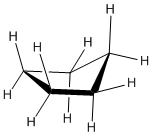3D structure of cyclopentane (notice that the far top right carbon is the endo position).

At room temperature, cyclopentane undergoes a rapid bond rotation process in which each of the five carbons takes turns being in the endo position.Cyclopentane distorts only very slightly into an "envelope" shape in which one corner of the pentagon is lifted up above the plane of the other four. The envelope removes torsional strain along the sides and flap of the envelope by allowing the bonds to be in an almost completely staggared position. However, the neighboring bonds are eclipsed along the "bottom" of the envelope, away from the flap. Viewing a Newman projections of cyclopentane signed down one of the C-C bond show the staggered C-H bonds.Newman projection of cyclopentane

• The angle strain in the envelope conformation of cyclopentane is low. The ideal angle in a regular pentagon is about 107o, very close to the preferred 109.5o tetrahedral bond angle.
• There is some torsional strain in cyclopentane. The envelope conformation reduces torsional strain by placing some bonds in nearly staggered positions. However, other bonds are still almost fully eclipsed.
• Cyclopentane is not large enough to allow for steric strain to be created.
• Overall, cyclopentane has very little ring strain (26 kJ/mol) when compared to cyclopropane and cyclobutane.

Interactive Element

The 3D Structure of Cyclopentane

## C3-C5 Cycloalkanes in Nature

If one of the carbon-carbon bonds is broken in cyclopropane or cyclobutane, the ring will ‘spring’ open, releasing energy as the bonds reassume their preferred tetrahedral geometry. The effectiveness of two antibiotic drugs, fosfomycin and penicillin, is due in large part to the high reactivity of the three- and four-membered rings in their structures.One of the most important five-membered rings in nature is a sugar called ribose –  DNA and RNA are both constructed upon ‘backbones’ derived from ribose. Pictured below is one thymidine (T) deoxy-nucleotide from a stretch of DNA. Since the ribose has lost one of the OH groups (at carbon 2 of the ribose ring), this is part of a deoxyribonucleic acid (DNA). If the OH at carbon 2 of the ribose ring was present, this would be part of a ribonucleic acid (RNA).The lowest-energy conformations for ribose are envelope forms in which either C3 or C2 are endo, on the same side as the C5 substituent.## Exercises

1) If cyclobutane were to be planar, how many H-H eclipsing interactions would there be? Assuming 4 kJ/mol per H-H eclipsing interaction what would the strain be on this “planar” molecule?

2) In the two conformations of trans-1,2-Dimethylcyclopentane one is more stable than the other. Explain why this is.3) In methylcyclopentane, which carbon would most likely be in the endo position?

## Solutions

1) There are 8 eclipsing interactions (two per C-C bond). The extra strain on this molecule would be 32 kJ/mol (4 kJ/mol x 8).

2) The first conformation is more stable. Even though the methyl groups are trans in both models, they are anti to one another in the first structure (which is lower energy) while they are gauche in the second structure increasing strain within the molecule.

3)  The ring carbon attached to the methyl group would most likely be the endo carbon.  The large methyl group would create the most torsional strain if eclipsed.  Being in the endo position would place the bonds is a more staggered position which would reduce strain.

#### Questions

Q4.4.1

If cyclobutane were to be planar how many H-H eclipsing interactions would there be, and assuming 4 kJ/mol per H-H eclipsing interaction what is the strain on this “planar” molecule?

Q4.4.2

In the two conformations of trans-cyclopentane one is more stable than the other. Explain why this is.#### Solutions

S4.4.1

There are 8 eclipsing interactions (two per C-C bond). The extra strain on this molecule would be 32 kJ/mol (4 kJ/mol x 8).

S4.4.2

The first conformation is more stable. Even though the methyl groups are trans in both models, in the second structure they are eclipsing one another, therefore increasing the strain within the molecule compared to the first structure where the larger methyl groups are anti to one another.